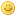Tetration is pentation. This deserve more thinking.marracoFellowPosts: 100 Threads: 12 Joined: Apr 2011 03/30/2015, 12:30 PM (This post was last modified: 03/31/2015, 02:36 PM by marraco.) Possibly iterated left bracket tetration has a way to solve real exponents of right bracket tetration. Here the bracket shows the place where, the result of the last iteration, is placed on the next iteration. Common tetration is right bracket, and the weak alternative is left bracket: $a\^\^{(m)}=a^{a^{a^{...}}}=\,^ma$ $(a)\^\^n={(((a)^a)^a ...)^a}=a^{a^{(n-1)}}=N_a\,^{{a^n}}$ where $\\[15pt] {N_a=a^{\frac{1}{a}}}$ Note that $\\[15pt] {(a)\^\^{(a+1)}=a^{{a}}=\,^2a}$ Let be $\\[15pt] {g(x)=N_a\,^{{a^{x+1}}}=a^{a^{x}}}$ And in general, n iterations: $\\[15pt] {f^{\circ n}\{g(a)\}=\,^{1+2n}a}\,\,\,\leftarrow\,\,\,$ this is actually a form of weak pentation, because is an iteration of a weak form of tetration. In some sense, tetration is a form of pentation, and we are trying to solve a pentation without understanding his base tetration. Note that if we solve this iteration for $\\[15pt] {n \in R}$, we also solve tetration to any real exponents.marracoFellowPosts: 100 Threads: 12 Joined: Apr 2011 03/30/2015, 12:43 PM (This post was last modified: 03/31/2015, 02:26 PM by marraco.) An alternative form is $\\[15pt] {g(x)=ln_{N_a}((a)\^\^{x})=a^{{x}}}$ then $\\[15pt] {f^{\circ n}\{g(a)\}=\,^{n}a}$ also: $\\[25pt] { ln_{N_m}( {(m)\^\^{\,^nm }} ) \,=\, {^{n+2}m}$marracoFellowPosts: 100 Threads: 12 Joined: Apr 2011 03/30/2015, 02:54 PM (This post was last modified: 03/30/2015, 04:14 PM by marraco.) Observe this incredible sequence $\\[15pt] {(a)\^\^a = a^{a-1} = N_a\,^{a^a} \,=\, N_a\,^{N_a\,^{a^2}} \,=\, N_a\,^{N_a\,^{N_a\,^{2a}}} \,=\, N_a\,^{N_a\,^{N_a\,^{N_a\,^{ln_{a}(2^a)+a}}}} \,=\, ... }$ This hints that the zeration $\\[15pt] {a\circ a=ln_{N_a}(2)+a }$, which is even another argument in favor of $\\[15pt] {a\circ b=ln_{?}(?^a+?^b) }$, but also hints about what is below zeration, and maybe it can explored over tetration. -Is trivial that the former sequence of towers converge to $\\[15pt] { a^{a-1} }$. -Is puzzling that the exponent (2+a) is missing. « Next Oldest | Next Newest »

 Possibly Related Threads… Thread Author Replies Views Last Post Pentation fractal Daniel 11 1,672 08/03/2022, 03:04 AM Last Post: JmsNxn pentation and hexation sheldonison 9 23,161 09/18/2019, 02:34 PM Last Post: sheldonison Mizugadro, pentation, Book Kouznetsov 41 103,333 03/02/2015, 08:13 PM Last Post: sheldonison Infinite Pentation (and x-srt-x) andydude 20 49,399 05/31/2011, 10:29 PM Last Post: bo198214 Regular "pentation"? mike3 12 36,734 04/04/2011, 03:16 AM Last Post: BenStandevenPentation roots self but please you do... nuninho1980 2 11,361 11/03/2010, 12:54 PM Last Post: nuninho1980 Pentation's definitional ambiguity Base-Acid Tetration 14 39,533 12/15/2009, 11:23 PM Last Post: Base-Acid Tetration Complex fixed points of base-e tetration/tetralogarithm -> base-e pentation Base-Acid Tetration 19 54,374 10/24/2009, 04:12 AM Last Post: andydude Exploring Pentation - Base e jaydfox 22 62,864 03/03/2008, 08:04 PM Last Post: Ivars

Users browsing this thread: 1 Guest(s)